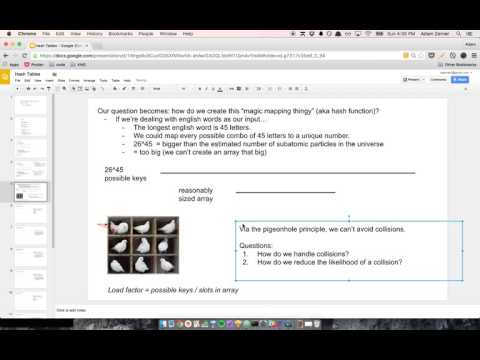Big O, little n

I remember when I was first learning about hash tables. I thought I stumbled across an ingenious optimization for dealing with hash collisions. Before explaining my optimization, let me first briefly explain how hash tables work and what hash collisions are. I also made a video if you're interested.Say that we have a hash that maps someone's name to their age. "adam" is 27, so we want to enter that into our hash.Here's what happens:

• "adam" gets put through a hash function.
• The hash function spits out 7.
• 7 is the index of the array where we are going to store "adam"'s value.
• So we store 27 into array.

If we run hash.get("adam") in the future and need to look up the value for "adam", we:

• Run "adam" through the hash function.
• Get 7 as the output.
• This means we have to look at array to get the value.
• So we look at array to get our value.

Hopefully if we have a key of "alice" or "bob" it'll hash to a different index. But... what happens if it hashes to the same index? That's called a hash collision.A common approach is that if there's a collision, you store the values in a linked list.Fair enough. That makes sense.

But wait!!! It takes O(n) time to look up a value in a linked list. Can't we use a binary search tree to speed that up to O(log n)???Or... can't we take it a step further and use a hash inside of a hash to get the lookup time all the way down to O(1)?!I hate to say it, but however many years ago when I had these thoughts, there were a few moments where I thought I was a genius. Now when I look back at that former self, I facepalm.

It is true that going from a linked list to a BST to a hash takes you from O(n) to O(logn) to O(1) lookup time. However, since collisions are rare, n is going to be small. And when n is small, O(n) might be faster than O(1).

To understand why that is, think back to what Big-O really means. I think it helps to think about it as a "math thing" instead of a "programming thing". Consider two functions:

f(n) = 4n + 1000
g(n) = 2n^2 + 5

Big-O of f is O(n) and Big-O of g is O(n^2). We just focus on the part that "really matters". When n gets really big, the fact that it's 4n doesn't really matter. Nor does the + 1000.

But what about when n is small? Well, let's look at happens when n is 5:

f(5) = 4(5) + 1000 = 20 + 1000 = 1020
g(5) = 2(5^2) + 5 = 2(25) + 5 = 50 + 5 = 55

Look at that! The O(n^2) function is almost 20 times faster than the O(n) function!

And that is basically why they use a linked list instead of a BST or hash to handle collisions. Since n is small, Big-O isn't the right question to ask.

Here's another example. I just wrote the following code while prepping for an interview:

const isVowel = (char) => ["a", "e", "i", "o", "u"].includes(char);

Normally I wouldn't think twice about it, but since interviewers care so much about time complexity, I stopped to think about whether it could be improved.

And it hit me that it's actually O(n)(caveat), because we've got an array and have to iterate over every element to see if the element matches char. It's easy to overlook this because we're using includes and not writing the code ourselves.

So then I thought that maybe we could use a hash instead to get O(1) lookup. Something like this:

const isVowel = (char) => {
const vowels = {
a: true,
e: true,
i: true,
o: true,
u: true,
};

return !!vowels[char];
};

But then I realized that this is the same mistake I made with the hash collision stuff however many years ago. n is small, so Big-O isn't the question we should be asking. Here n is 5.

I think that the array approach would actually be faster than the hash approach, even though it's O(n) instead of O(1). The reason for this is called locality.

If you dive deep under the hood, when you look up an element in an array, the CPU actually grabs a bunch of adjacent elements as well as the one you wanted, and it stores the adjacent elements in a cache. So here when we look up "a" in the array, it'll probably grab "a", "e", "i", "o", and "u". And so next time when we want to grab "e", it can take it from the cache, which is a lot faster. This works because the elements in the array are close to each other in the physical memory. But with a hash, my understanding is that they wouldn't be so close, and thus we wouldn't benefit from this spatial locality effect.

I'm no low-level programming wiz so I'm not sure about any of this. That's ok, I think it's beside the point of this post. The real point of this post is that when you have a little n, Big-O doesn't matter.

If you have any thoughts, I'd love to discuss them over email: adamzerner@protonmail.com.

If you'd like to subscribe, you can do so via email or RSS feed.

- 102 toasts